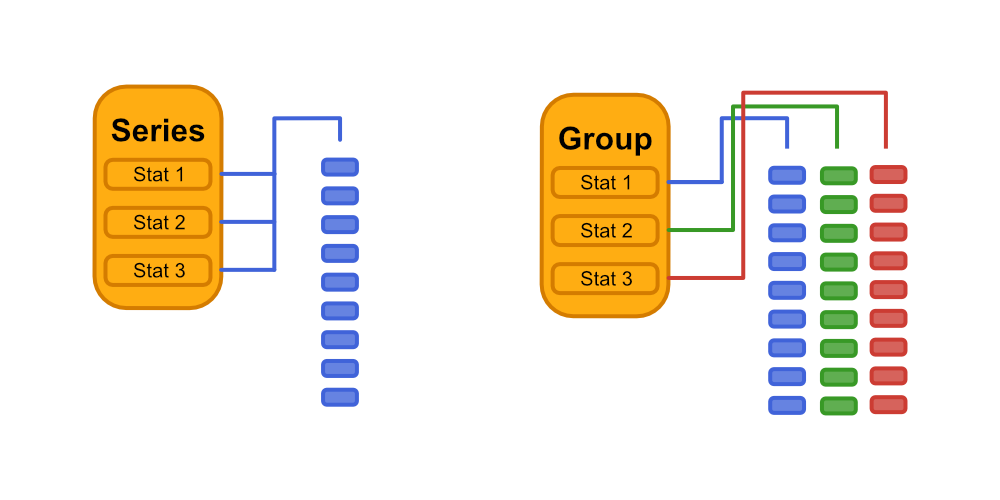Home

# Home

OnlineStats is a Julia package for statistical analysis with algorithms that run both online and in parallel. Online algorithms are well suited for streaming data or when data is too large to hold in memory. Observations are processed one at a time and all algorithms use O(1) memory.

## Installation

``````import Pkg

## Basics

### Every stat is `<: OnlineStat{T}`

(where `T` is the type of a single observation)

``````julia> using OnlineStats

julia> m = Mean()
Mean: n=0 | value=0.0

julia> supertype(typeof(m))
OnlineStat{Number}``````

### Stats can be updated

`fit!(stat::OnlineStat{T}, y::S)` will iterate through `y` and `fit!` each element if `T != S`.

``````julia> y = randn(100);

julia> fit!(m, y)
Mean: n=100 | value=-0.0442521``````

### Stats can be merged

``````julia> y2 = randn(100);

julia> m2 = fit!(Mean(), y2)
Mean: n=100 | value=-0.0885959

julia> merge!(m, m2)
Mean: n=200 | value=-0.066424``````

### Stats have a value

``````julia> value(m)
-0.06642399933674126``````

## Collections of Stats### `Series`

A `Series` tracks stats that should be applied to the same data stream.

``````y = rand(1000)
s = Series(Mean(), Variance())
fit!(s, y)``````
``````Series
├── Mean: n=1000 | value=0.511416
└── Variance: n=1000 | value=0.0829059``````

### `FTSeries`

An `FTSeries` tracks stats that should be applied to the same data stream, but filters and transforms (hence `FT`) the input data before it is sent to its stats.

``````s = FTSeries(Mean(), Variance(); filter = x->true, transform = abs)
fit!(s, -y)``````
``````FTSeries
├── Mean: n=1000 | value=0.511416
└── Variance: n=1000 | value=0.0829059``````

### `Group`

A `Group` tracks stats that should be applied to different data streams.

``````g = Group(Mean(), CountMap(Bool))
itr = zip(randn(100), rand(Bool, 100))
fit!(g, itr)``````
``````Group
├── Mean: n=100 | value=-0.0879653
└── CountMap: n=100 | value=OrderedCollections.OrderedDict(true=>53,false=>47)``````